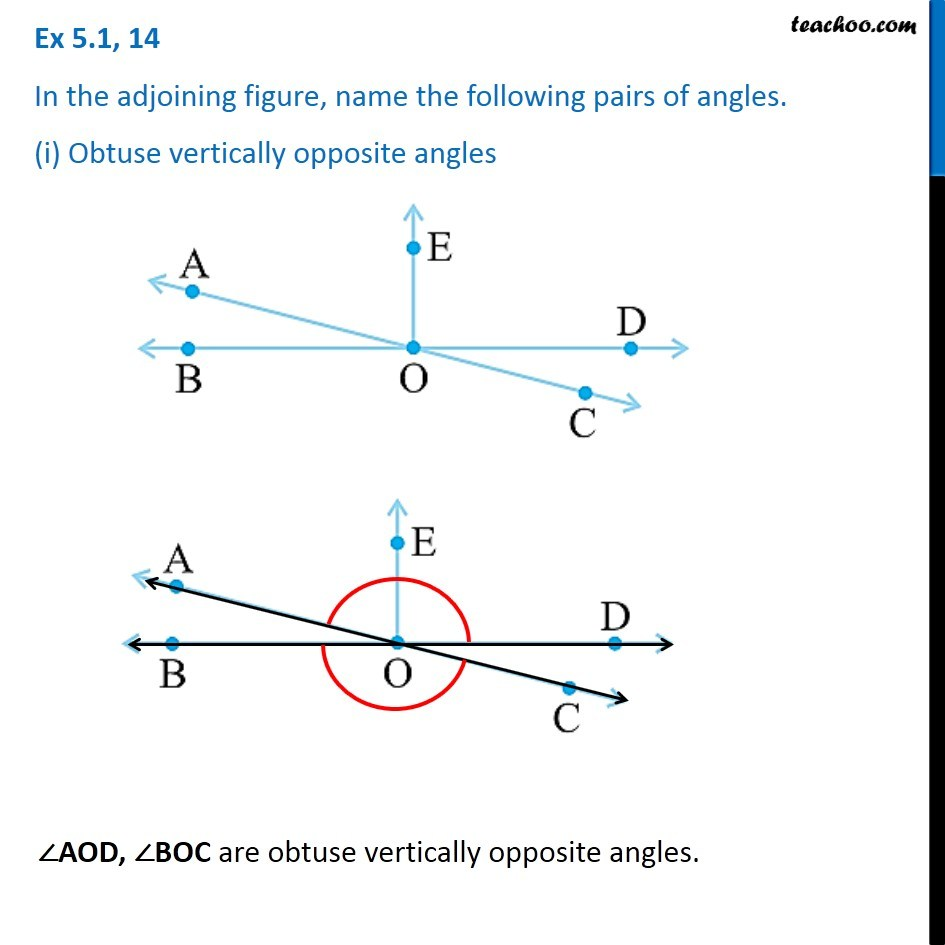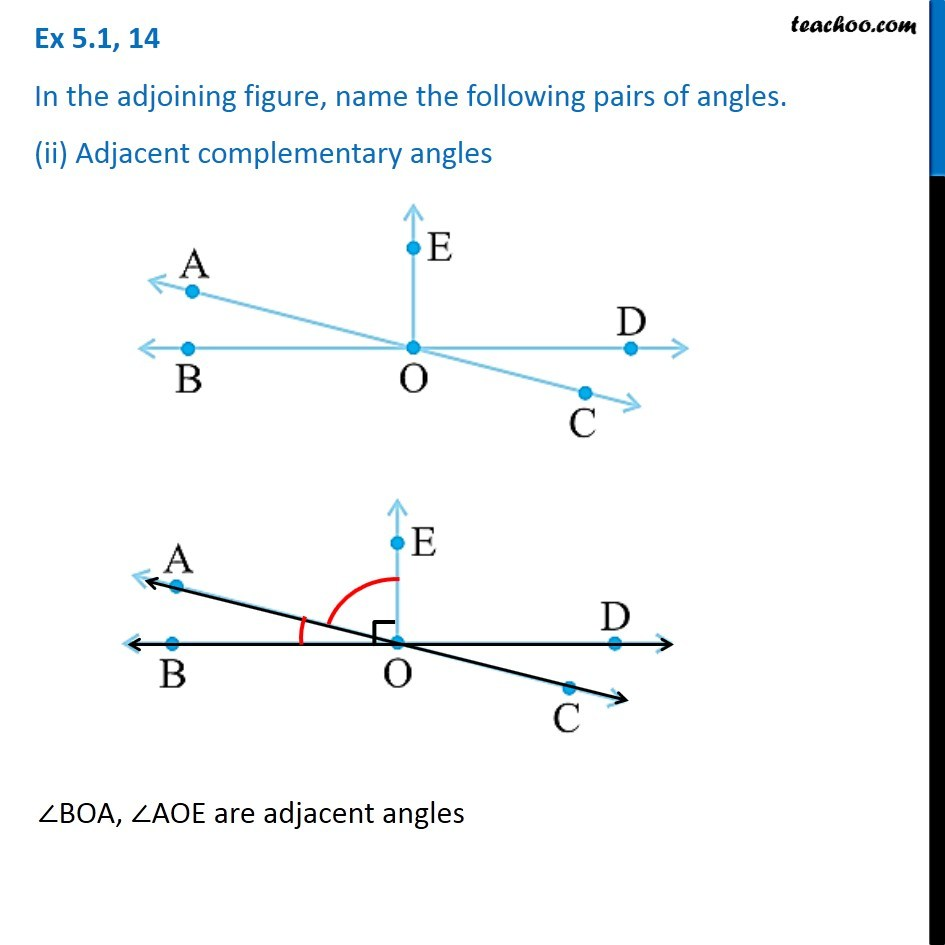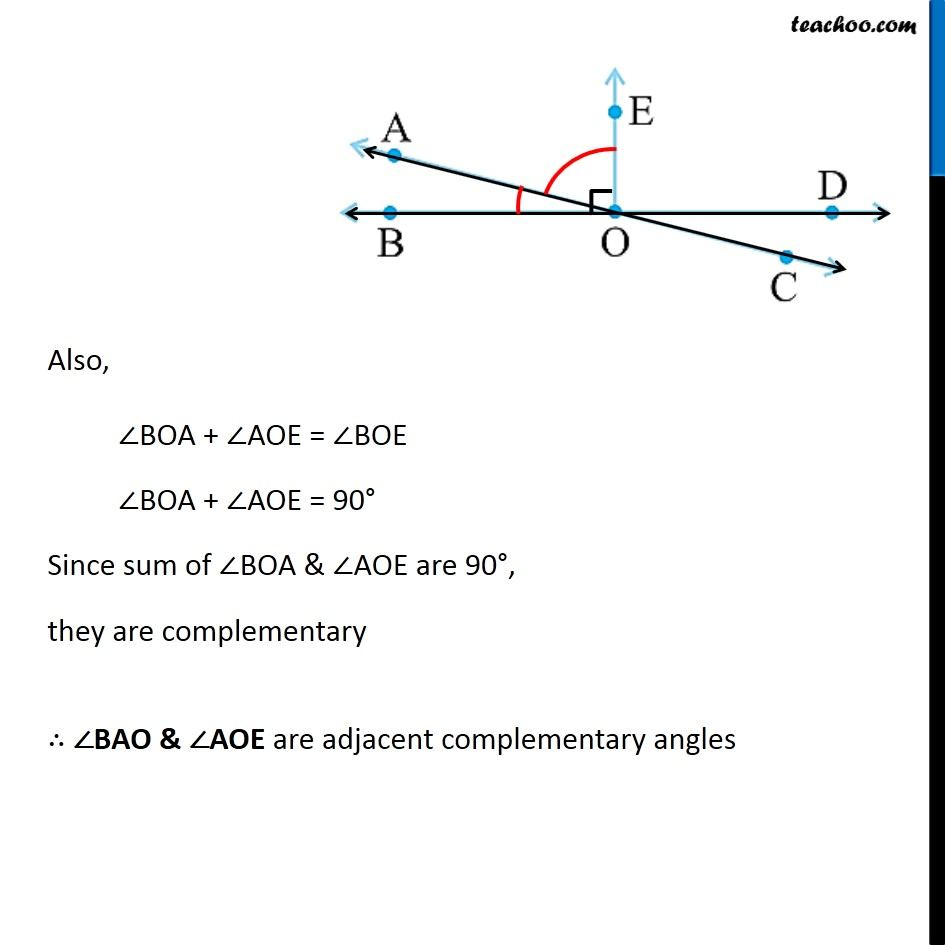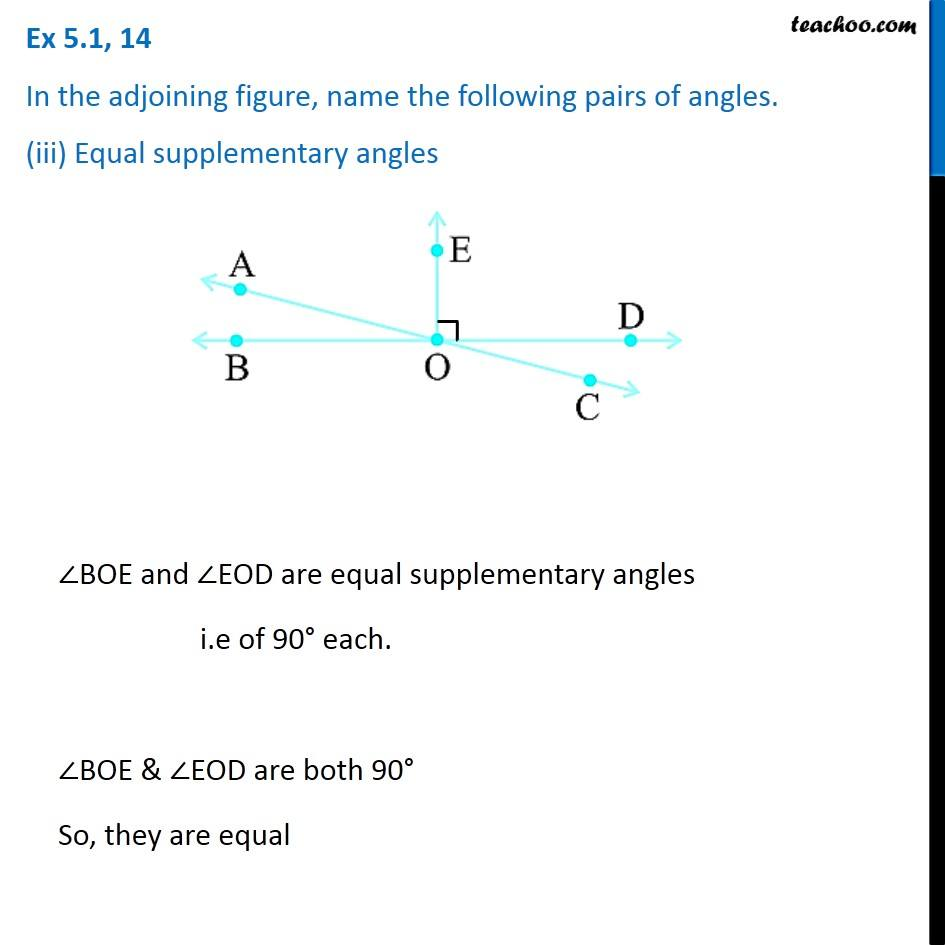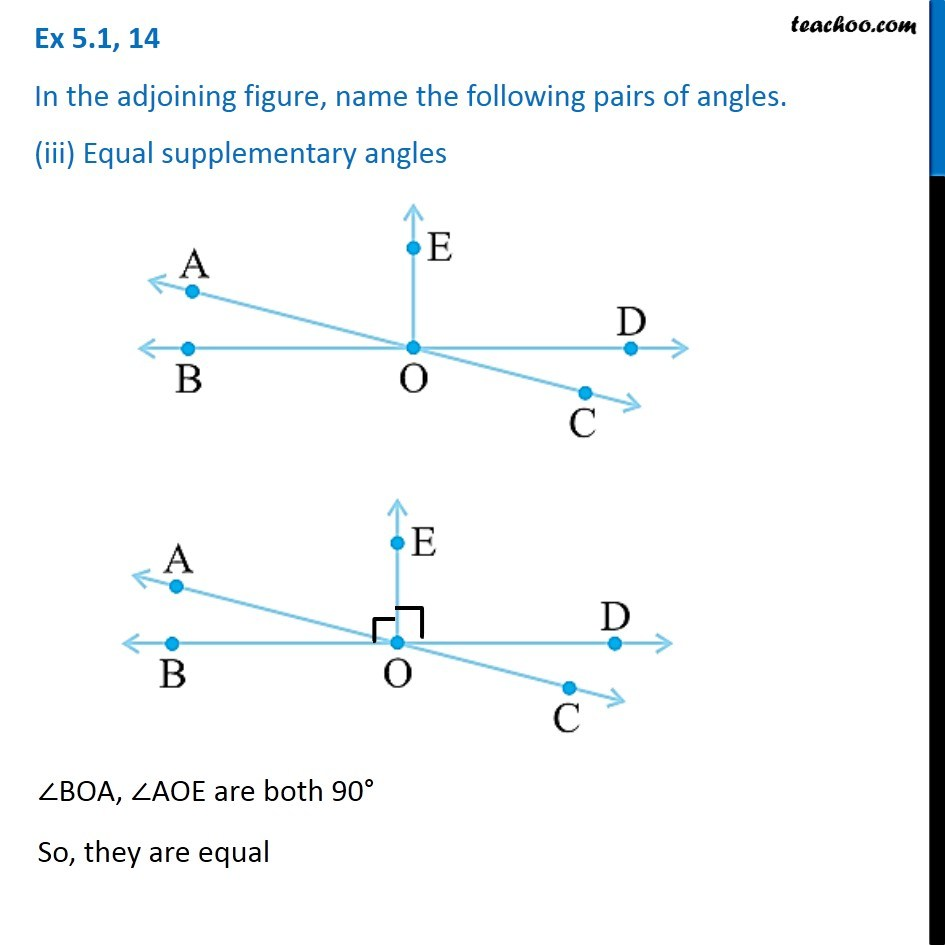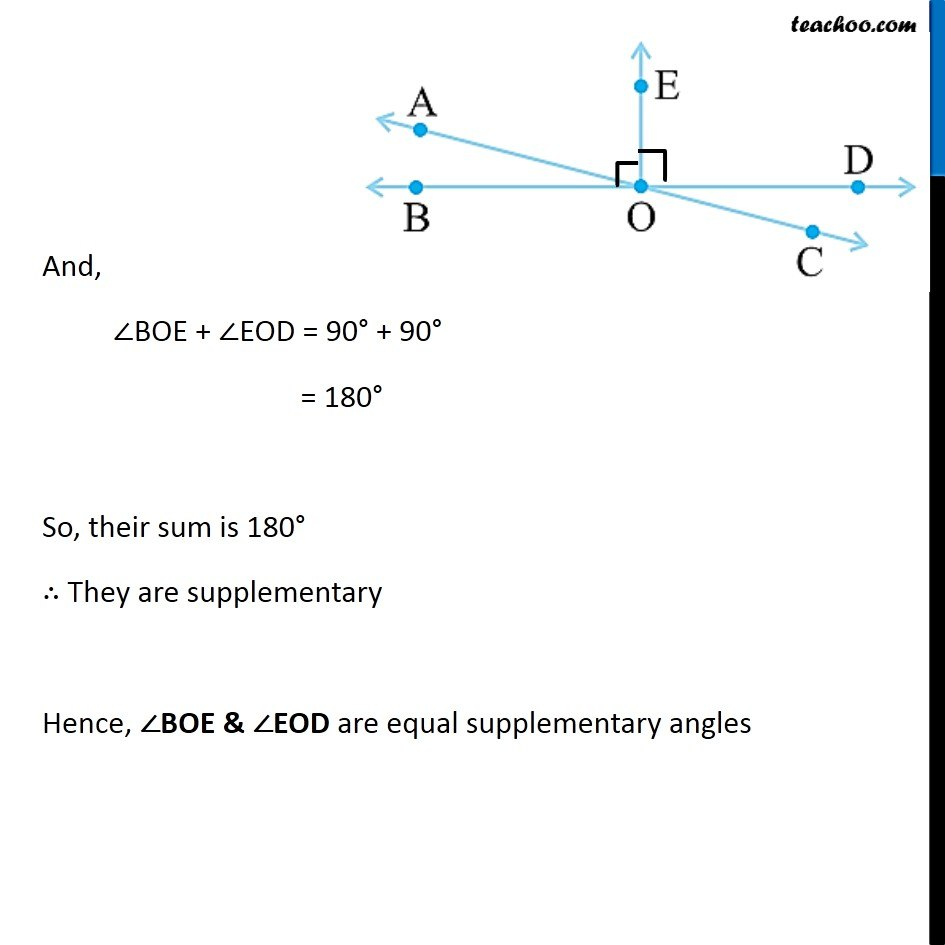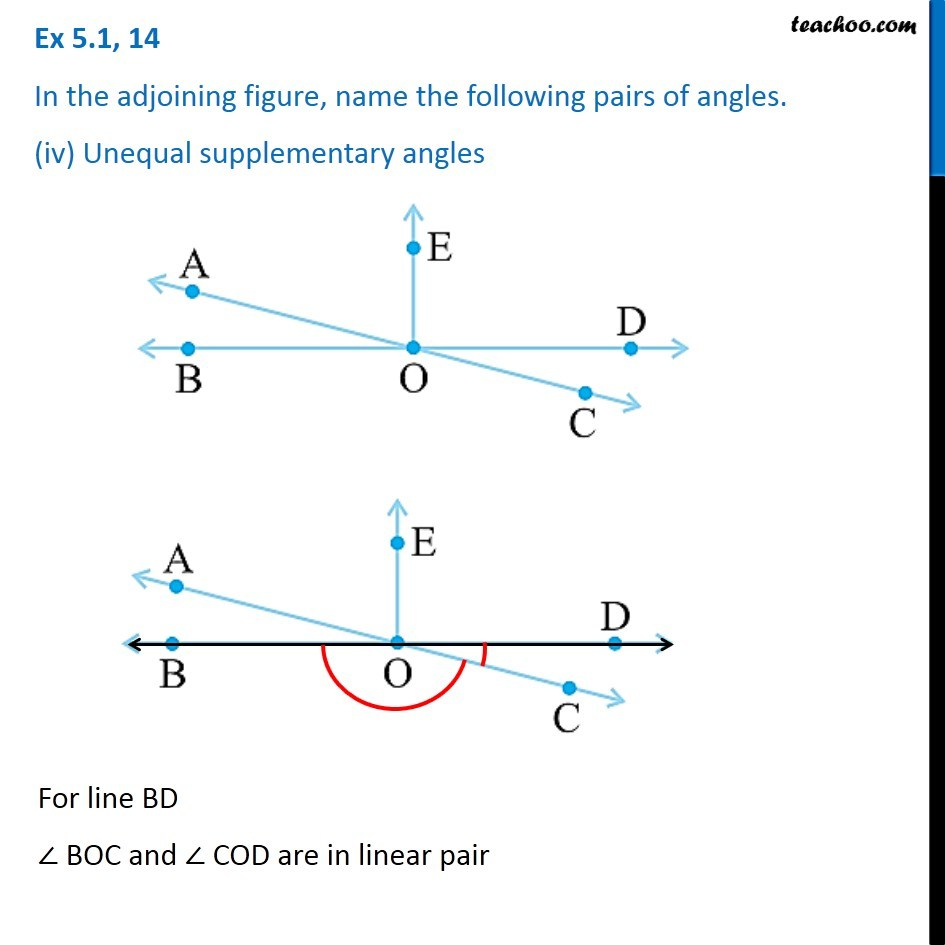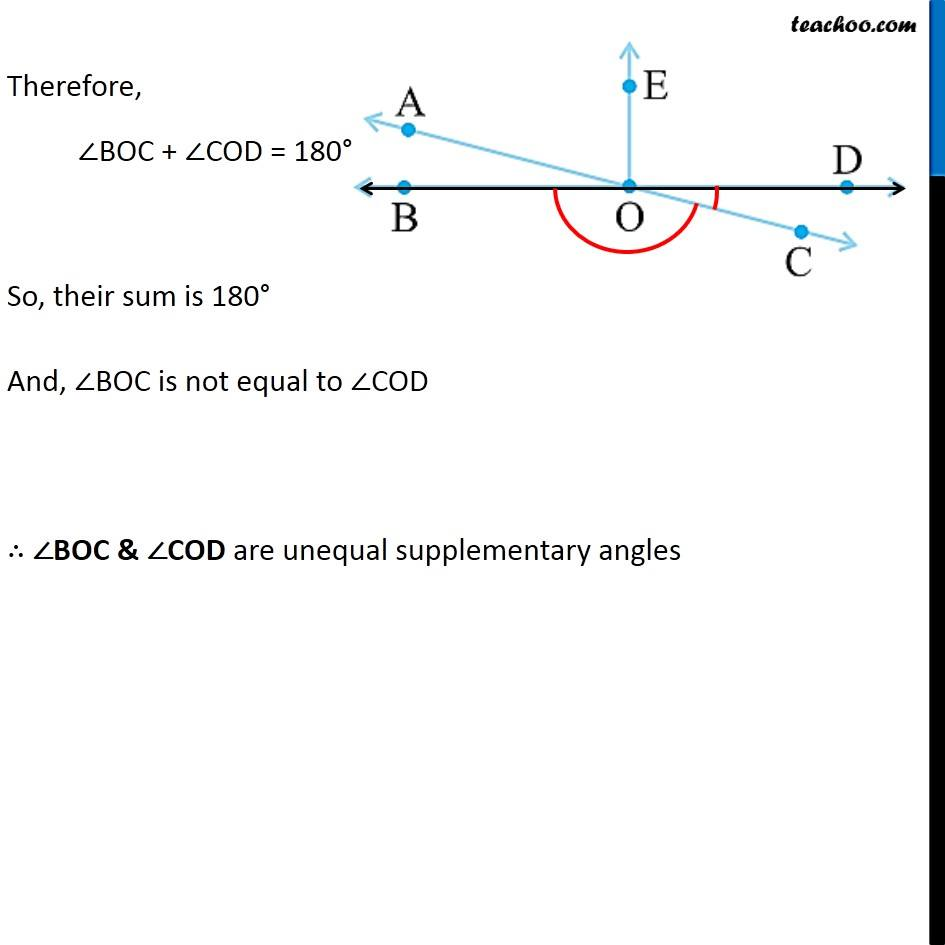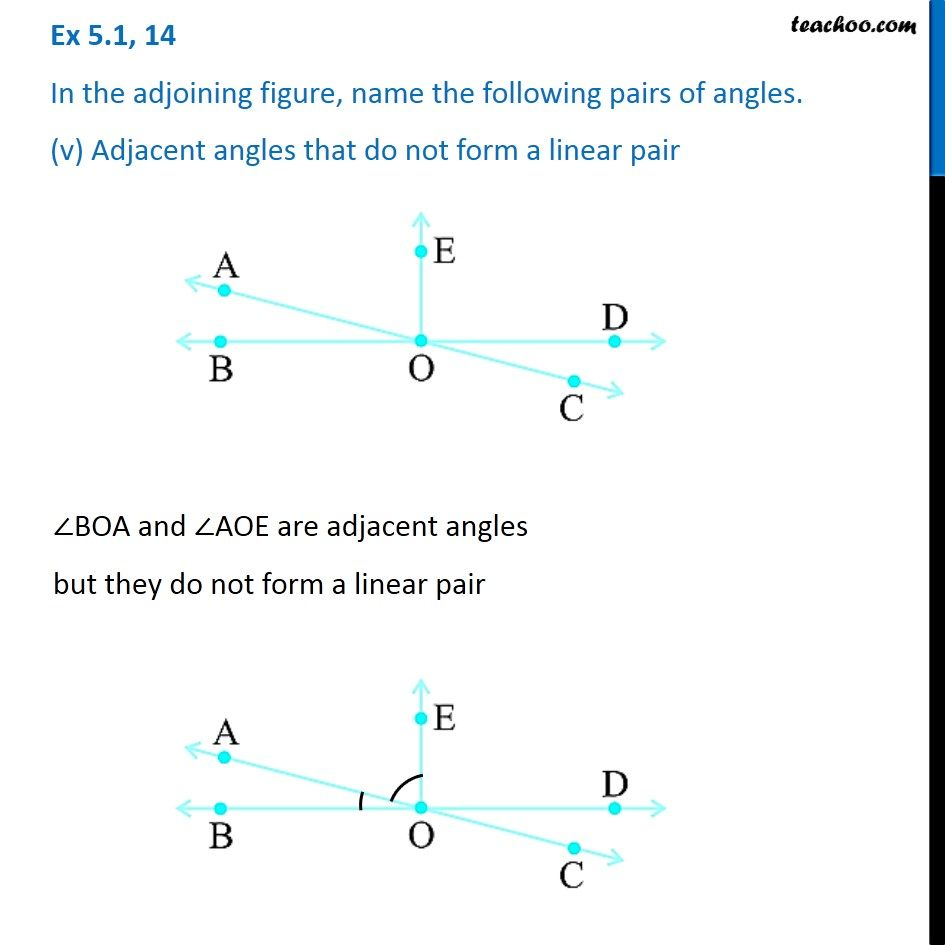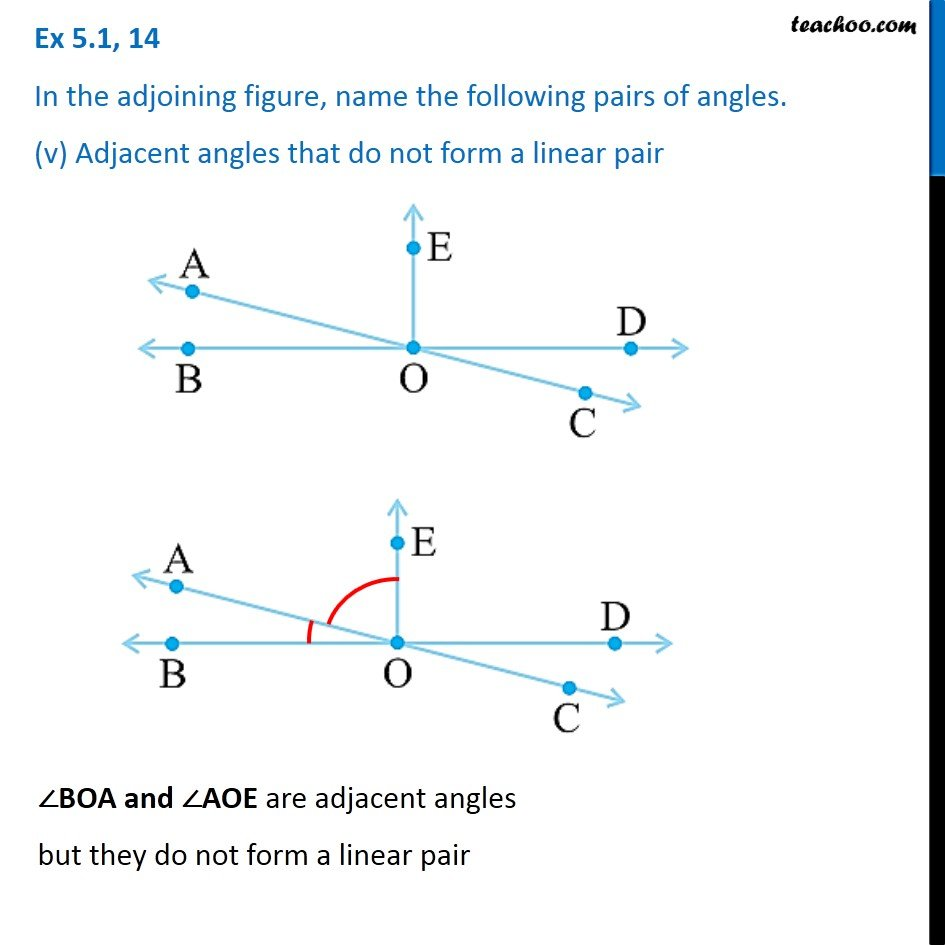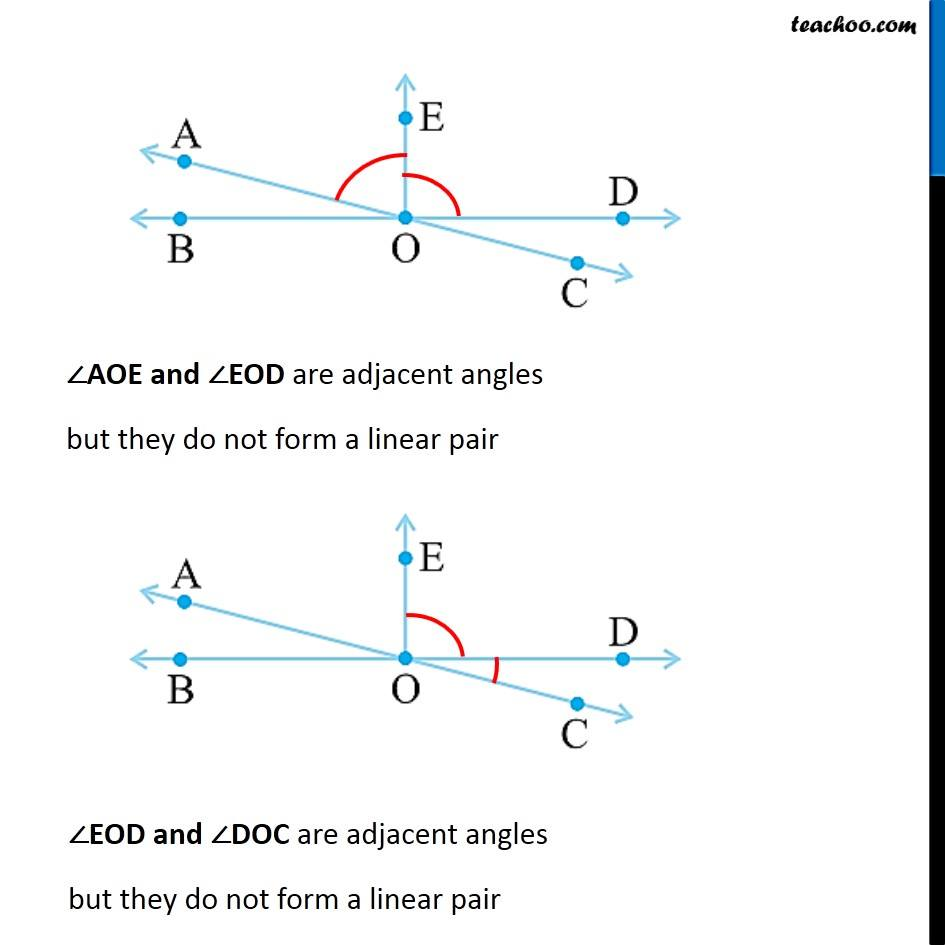1. Chapter 5 Class 7 Lines and Angles
2. Concept wise
3. Adjacent Angles, Linear Pair of angles, Vertically Opposite angles

Transcript

Ex 5.1, 14 In the adjoining figure, name the following pairs of angles. (i) Obtuse vertically opposite angles∠AOD, ∠BOC are obtuse vertically opposite angles. Ex 5.1, 14 In the adjoining figure, name the following pairs of angles. (ii) Adjacent complementary angles∠BOA, ∠AOE are adjacent angles Also, ∠BOA + ∠AOE = ∠BOE ∠BOA + ∠AOE = 90° Since sum of ∠BOA & ∠AOE are 90°, they are complementary ∴ ∠BAO & ∠AOE are adjacent complementary angles Ex 5.1, 14 In the adjoining figure, name the following pairs of angles. (iii) Equal supplementary angles∠BOE and ∠EOD are equal supplementary angles i.e of 90° each. ∠BOE & ∠EOD are both 90° So, they are equal Ex 5.1, 14 In the adjoining figure, name the following pairs of angles. (iii) Equal supplementary angles ∠BOA, ∠AOE are both 90° So, they are equal And, ∠BOE + ∠EOD = 90° + 90° = 180° So, their sum is 180° ∴ They are supplementary Hence, ∠BOE & ∠EOD are equal supplementary angles Ex 5.1, 14 In the adjoining figure, name the following pairs of angles. (iv) Unequal supplementary anglesFor line BD ∠ BOC and ∠ COD are in linear pair Therefore, ∠BOC + ∠COD = 180° So, their sum is 180° And, ∠BOC is not equal to ∠COD ∴ ∠BOC & ∠COD are unequal supplementary angles Ex 5.1, 14 In the adjoining figure, name the following pairs of angles. (v) Adjacent angles that do not form a linear pair∠BOA and ∠AOE are adjacent angles but they do not form a linear pair Ex 5.1, 14 In the adjoining figure, name the following pairs of angles. (v) Adjacent angles that do not form a linear pair ∠BOA and ∠AOE are adjacent angles but they do not form a linear pair ∠AOE and ∠EOD are adjacent angles but they do not form a linear pair ∠EOD and ∠DOC are adjacent angles but they do not form a linear pair

Adjacent Angles, Linear Pair of angles, Vertically Opposite angles